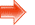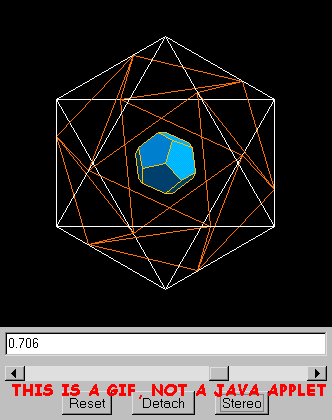# Morphing PolyhedronMorphing Polyhedron Applet (below) doesn't work even though you installed Java? Run the Morphing Polyhedron Web-Start Application instead. This downloads a jnlp (Java Web Start) file that tells Java how to run the Morphing Polyhedron outside of your browser. See my Java Web Start notes.More Java applets here.

This is a Java 1.1 applet. See my Java page for Browser Requirements.

### Overview

If you familiarize yourself with the way this thing works, you'll always be able to produce icosahedron vertex coordinates all by yourself (without having to look them up in a book). This can be handy if you're stranded on a desert island. I personally used this algorithm at a halloween pumpkin-carving party to mark up a pumpkin in an icosahedral pattern.

Initially (or after clicking the Reset button) you see a solid Icosahedron inscribed in a wireframe Octahedron. The vertices of the Icosahedron lie on the edges of the Octahedron.

You can morph the polyhedron by adjusting the slider control.

You can rotate the image by dragging with the mouse.

The "text box" above the slider shows its setting, which varies from 0.0 to 1.0 in steps of 0.001. (The slider is a "native" control, which is known to have peculiar behaviors on certain platforms -- you may not be able to adjust it all the way to 1.0.)

By adjusting the slider, you can see these polyhedra:

 Polyhedron Slider Setting Exact Octahedron 0.0 0 Icosahedron 0.382 1/(1+G) Cuboctahedron 0.5 1/2 Regular Dodecahedron 0.618 G/(1+G) Rhombic Dodecahedron 0.667 2/3 Regular Dodecahedron 0.724 (1+G)/(2+G) Tiny cube Approaching 1.0 Approaching 1.0

Where G is the golden ratio: (1+sqrt(5))/2.

### Explanation, for Slider Settings <=0.5

A point is selected on each edge of the wire-frame Octahedron. The slider controls the placement of these points along the edge (as a fraction of total edge-length).

On each of the 8 faces of the Octahedron, we connect the three selected edge-points to form an equilateral triangle (green).

Twelve isoceles triangles (blue) are made by connecting edge-points from adjacent Octrahedron faces (two blue triangles under each of the 6 vertices).

The total number of triangles is 20 (8 green equilateral and 12 blue isoceles).

For a particular slider setting (approximately 0.382), the blue triangles become equilateral (and thus equal to the green triangles) and an icosahedron is formed.

At this particular slider setting, the edge is divided into a larger and smaller part whose lengths are related by the Golden Ratio.

This method of constructing the icosahedron is useful because it provides a quick way to calculate icosahedron vertex coordinates if you know octahedron vertex coordinates. Octahedron vertex coordinates are easy to write down. Here are vertices for an octahedron of edge length sqrt(2):
( 1, 0, 0)
(-1, 0, 0)
( 0, 1, 0)
( 0,-1, 0)
( 0, 0, 1)
( 0, 0,-1)

If the slider is set to 0.5, the blue triangles become right-angled, and pairs of them merge to form the six square faces of a Cuboctahedron.

### Explanation, for Slider Settings >0.5

The behavior in this slider range was totally unexpected -- a pleasant surprise.

Understanding what is happening here is a little tricky. I have to explain what the applet is actually doing. It uses a method of "slicing" that is a spin-off from my Hyperspace Polytope Slicer. The blue surfaces are made by slicing the Octahedron with infinite planes. These planes are defined by triplets of selected edge-points, as described above.

For slider settings <0.5 (described above), the effect of the slicing is easy to see because the slices create the blue triangles whose corners are the selected edge-points that define the slicing plane. Each pair of slicing planes meets in a raised edge under an Octahedron vertex.

When the slider setting reaches 0.5, that edge is no longer raised. In fact there is no edge because the pair of slicing planes coincide.

As the slider setting is increased above 0.5, the edge where the planes meet is depressed rather than raised. The two planes undercut each other and the solid figure detaches from the Octahedron edges. You can see this most clearly if you rotate the figure so that you are looking directly down on a vertex of the Octahedron.

Note that when the solid figure has detached from the Octahedron edges, the applet draws orange lines on the Octahedron surface to indicate the locations of the selected points.

An exercise for the obsessive-compulsive reader: try proving all the slider settings tabulated above. To do this, you need to know that the regular icosahedron and the regular dodecahedron are "duals". They can be formed from each other by interchanging faces and vertices. For example, if you draw radial lines from the center of the icosahedron to all 12 of its vertices, and place planes perpendicular to those lines, all equidistant from the center, the figure that is enclosed by those planes is a dodecahedron. You also need to know that a rhombic dodecahedron can be formed from an octahedron in a somewhat similar way. In this case, you draw radial lines from the center of the octahedron to the centers of its 12 edges, and place planes perpendicular to those lines, all equidistant from the center.

• Slider
Adjust by dragging. Single-step by clicking the button on the left or right.
• "Reset" Button
Resets to the initial slider setting and rotation angle.
• "Detach" Button
Detaches the applet into its own window which can be resized. The button label changes to say "Attach".
• "Stereo" Button
Toggles between a single image and a stereoscopic double-image, to be viewed with the "look crossed" method.
• Image panel
Drag with the mouse to rotate the image.

### Acknowledgement

I got this method of constructing the icosahedron and cuboctahedron from H.S.M. Coxeter's book "Regular Polytopes" (Coxeter01).

Coxeter credits P. Schönemann for the method (1873). (Schonemann01)